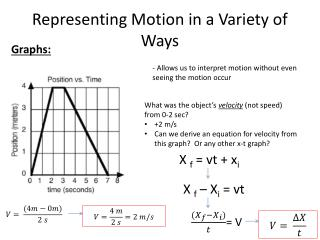DownloadDownload PresentationRepresenting Motion in a Variety of Ways

# Representing Motion in a Variety of Ways

Download Presentation## Representing Motion in a Variety of Ways

- - - - - - - - - - - - - - - - - - - - - - - - - - - E N D - - - - - - - - - - - - - - - - - - - - - - - - - - -
##### Presentation Transcript

1. Representing Motion in a Variety of Ways Graphs: - Allows us to interpret motion without even seeing the motion occur • What was the object’s velocity (not speed) from 0-2 sec? • +2 m/s • Can we derive an equation for velocity from this graph? Or any other x-t graph? X f = vt + xi X f – Xi = vt = V

2. Position-Time Graphs (x-t) Velocity from 2-3 seconds? Velocity from 3-7 seconds? =

3. Motion Maps • Another way to represent an object’s motion • We can go back and forth between graphs and motion maps to represent the same motion • To make a motion map, you need to draw an arrow representing positive direction Constant positive velocity X Beginning of the arrow is the reference point (origin) • If the object is moving, its position will change over time. If the object is stationary, its position will remain the same as time increases • On a motion map, this is illustrated by a single dot on the position arrow

4. Motion Maps Object is stationary X • An object’s constant velocity is indicated by 2 things: • The displacement between the points are constant (change in position is equal) • Arrows indicating the object’s velocity are the same length Constant positive velocity X If the object is stationary, then the velocity is zero and there is not an arrow indicating velocity X

5. More than one object • How do we know which object is traveling faster? • slope: remember, slope = velocity • Steeper the slope, faster the velocity B X (m) A Object B has a greater displacement (change in position) than A IN THE SAME AMOUNT OF TIME 2 t (sec)

6. Review from yesterday Y-intercept = starting position Slope = Velocity IS NOT THE SAME as speed • Speed • How fast • Velocity • How fast • direction X X

7. Consider the following x-t graphs • Rank these situations from greatest to least based on which shows the greatest displacement during the time from 0 to 10 sec. Use the > and = signs, but do not use the < sign. • Rank these situations from greatest to least based on which shows the greatest distance traveled during the time from 0 to 10 sec. Use the > and = signs, but do not use the < sign.